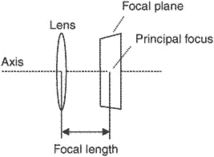# focal plane

(redirected from focal planes)
Also found in: Dictionary, Medical.

## focal plane

See focal length.
Collins Dictionary of Astronomy © Market House Books Ltd, 2006

## focal plane

[′fō·kəl ‚plān]
(optics)
A plane perpendicular to the axis of an optical system and passing through the focal point of the system.
McGraw-Hill Dictionary of Scientific & Technical Terms, 6E, Copyright © 2003 by The McGraw-Hill Companies, Inc.

## focal planeThe plane occupied by the film at the focal point of the lens. It can be positive or negative; it is commonly referred to as positive and negative planes, respectively.
References in periodicals archive ?
Subsequently, the system matrix is assembled with the depth conversion matrices and a chosen focal plane. Finally, the system equation is solved with the Levenberg-Marquardt method to achieve the restored image.
For all the image restoration results, the depth of focal plane (DFP) was set as 2 cm, the voxel size was set as 0.05 cm, and the optical coefficients [u.sub.a] and [u'.sub.s] were set as 0.02 [cm.sup.-1] and 10 [cm.sup.-1], respectively.
As referred in the derivation of (10), the fluorescent signals are conceived to come from the focal plane. Consequently, if the focal plane is not located at the true depth of target, the image restoration will result in a virtual target in the focal plane and the location of this virtual target varies with DFP.
The primary contribution of this work is the construction of system matrix, which is achieved through the definition of a new unknown parameter and depth conversion matrices with a chosen focal plane. The new unknown parameter is defined through the first mean value theorem for definite integrals and represents a weighted average of the fluorescent yields along the detection direction.
5(a) and (c) show 2D images on the focal planes [z.sub.f] = 0 and [z.sub.f] = 0.2 m, respectively.
should be pixel displacements between the 2D images of two passes on the same focal planes. In Section 2.3, although only single pass CSAR data is used, there are pixel displacements between two 2D images on different focal planes.
For each subaperture, the height of target is reconstructed through the relationship between the pixel displacements in the image pair of two observations and the pixel displacements in the image pair of one observation on two different focal planes, and then 3D target coordinates can be obtained based on the retrieved height information and the 2D image coordinates.
When the focal plane [z.sub.f] is chosen as the height z of the target P(x, y, z), i.e., [z.sub.f] = z, the 2D image about the target is a focused point; otherwise, when [z.sub.f] [not equal to] z, the result shall be a defocused ring.
It also offers an opportunity for improved baffling in the form of a field stop near the projected focal plane.
If the original focal plane (the object) and resulting focal plane are equidistant from the relay lens (as shown in the diagram below), then the lens simply transfers the image from one focal plane to another without changing the system's effective focal length.
Since we will want to illuminate more than just the exact center of the focal plane, we add something like a half inch to this figure for a nice fully illuminated low-power field of view.

Site: Follow: Share:
Open / Close